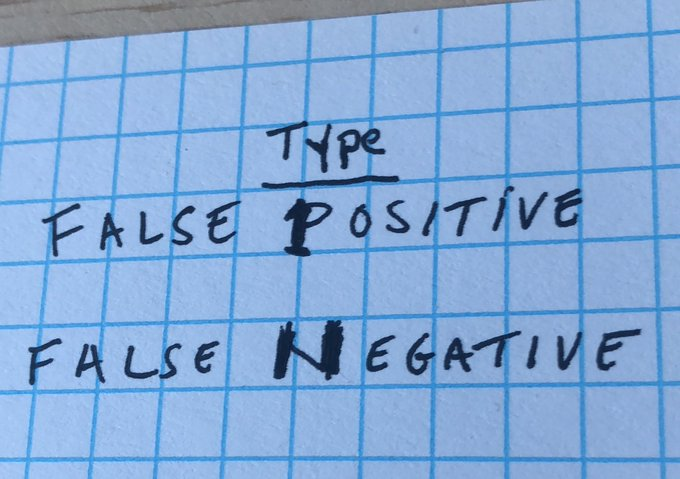# 7.7. Evaluating models¶

On the cross validation page, we said you choose the model that produces the “lowest average validation error” when you do your cross validation tests.

But wait! How do you measure accuracy of predictions or the model’s fit?

You need to pick a “scoring metric”!

Below, I list the most common statistics to report and consider. scikit-learn’s documentation lists the full menu.

Measurement name

Measures

Useful for predicting

R2

The fraction of variation in the y variable the model explains

Continuous variables

Root Mean Squared Error

Size of average squared error. 0 is impossible.

Continuous variable

Mean Absolute Error

Square errors penalize big prediction errors more. So MAE based models penalize these errors less. Intuitively, this means outliers influence the model less.

Continuous variable

True Positive Rate (TPR) aka “Sensitivity” aka “Recall” = 1-FNR

What fraction of the true positives do you call positive?

Binary variables

True Negative Rate (TNR) aka “Specificity” = 1-FPR

What fraction of the true negatives do you call negative?

Binary variables

“Precision”

How precise are your positive labels?
What fraction of what you labeled positive are truly positive?

Binary variables

“Accuracy”

What fraction of predictions made are correct?

Binary variables

False Positive Rate (FPR) = 1-TNR

What fraction of the true negatives do you call positive?

Binary variables

False Negative Rate (FNR) = 1-TPR

What fraction of the true positives do you call negative?

Binary variables

“F-score” or “F1”

The harmonic mean of recall and precision. Intuition: Are you detecting positive cases, and correctly?

Binary variables

Here is a great trick from Sean Taylor for remembering which errors are type 1 and type 2 (II):Which measurement should you use?

It depends! If you have a continuous variable, use R2, MAE, or RMSE.

If you have a classification problem (the “binary variable” rows in the table above), you have to think about your problem. I REALLY like the discussion on this page from DS100.

• In medical tests more broadly, false negatives are really bad - if someone has cancer, don’t tell them they are cancer free. Thus, medical tests tend to minimize false negatives.

• Minimizing false negatives is the same as maximizing the true positives, aka the “sensitivity” of a test, since $$FNR=1-TPR$$. Better covid tests have higher specificity, because if someone has covid, we want to know that so we can require a quarantine.

• In the legal field, false positives (imprisoning an innocent person) are considered worse than false negatives.

• Identifying terrorists? You might want to maximize the detection rate (“recall”). Of course, simply saying “everyone is a terrorist” is guaranteed to generate a recall rate of 100%! But this rule would have a precision of about 0%! So you also should think about “precision” too. Maybe F1 is the metric for you.